Advertisement Remove all ads

# NCERT solutions for Class 11 Physics Textbook chapter 9 - Mechanical Properties of Solids [Latest edition]

#### Chapters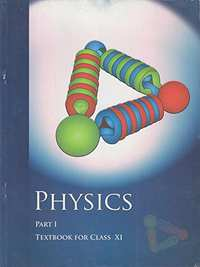Advertisement Remove all ads
Advertisement Remove all ads
Advertisement Remove all ads

## Chapter 9: Mechanical Properties of Solids

Advertisement Remove all ads
[Pages 242 - 245]

### NCERT solutions for Class 11 Physics Textbook Chapter 9 Mechanical Properties of Solids[Pages 242 - 245]

Q 1 | Page 242

A steel wire of length 4.7 m and cross-sectional area 3.0 × 10–5 m2 stretches by the same amount as a copper wire of length 3.5 m and cross-sectional area of 4.0 × 10–5 m2 under a given load. What is the ratio of Young’s modulus of steel to that of copper?

Q 2 | Page 242

The figure shows the strain-stress curve for a given material. What are (a) Young’s modulus and (b) approximate yield strength for this material?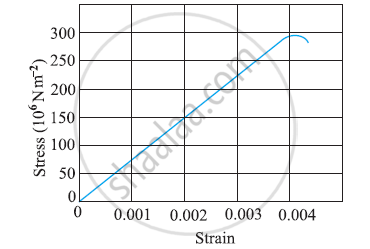Q 3 | Page 243

The stress-strain graphs for materials A and B are shown in Figure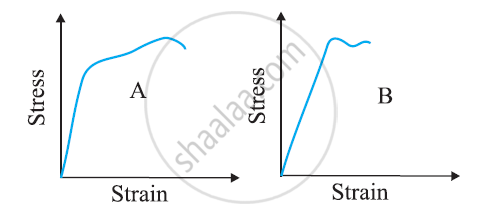The graphs are drawn to the same scale.

(a) Which of the materials has the greater Young’s modulus?

(b) Which of the two is the stronger material?

Q 4.1 | Page 243

Read the following statements below carefully and state, with reasons, if it is true or false

The Young’s modulus of rubber is greater than that of steel;

Q 4.2 | Page 243

Read the following statements below carefully and state, with reasons, if it is true or false.

The stretching of a coil is determined by its shear modulus.

Q 5 | Page 243

Two wires of diameter 0.25 cm, one made of steel and the other made of brass are loaded as shown in Fig. 9.13. The unloaded length of steel wire is 1.5 m and that of brass wire is 1.0 m. Compute the elongations of the steel and the brass wires.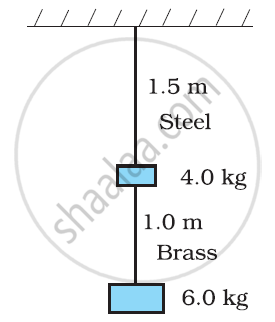Q 6 | Page 244

The edge of an aluminium cube is 10 cm long. One face of the cube is firmly fixed to a vertical wall. A mass of 100 kg is then attached to the opposite face of the cube. The shear modulus of aluminium is 25 GPa. What is the vertical deflection of this face?

Q 7 | Page 244

Four identical hollow cylindrical columns of mild steel support a big structure of mass 50,000 kg. The inner and outer radii of each column are 30 cm and 60 cm respectively. Assuming the load distribution to be uniform, calculate the compressional strain of each column.

Q 8 | Page 244

A piece of copper having a rectangular cross-section of 15.2 mm × 19.1 mm is pulled in tension with 44,500 N force, producing only elastic deformation. Calculate the resulting strain?

Q 9 | Page 244

A steel cable with a radius of 1.5 cm supports a chairlift at a ski area. If the maximum stress is not to exceed 10N m–2, what is the maximum load the cable can support?

Q 10 | Page 244

A rigid bar of mass 15 kg is supported symmetrically by three wires each 2.0 m long. Those at each end are of copper and the middle one is of iron. Determine the ratio of their diameters if each is to have the same tension.

Q 11 | Page 244

A 14.5 kg mass, fastened to the end of a steel wire of unstretched length 1.0 m, is whirled in a vertical circle with an angular velocity of 2 rev/s at the bottom of the circle. The cross-sectional area of the wire is 0.065 cm2. Calculate the elongation of the wire when the mass is at the lowest point of its path.

Q 12 | Page 244

Compute the bulk modulus of water from the following data: Initial volume = 100.0 litre, Pressure increase = 100.0 atm (1 atm = 1.013 × 105 Pa), Final volume = 100.5 litre. Compare the bulk modulus of water with that of air (at constant temperature). Explain in simple terms why the ratio is so large.

Q 13 | Page 244

What is the density of water at a depth where the pressure is 80.0 atm, given that its density at the surface is 1.03 × 103 kg m–3?

Q 14 | Page 244

Compute the fractional change in volume of a glass slab, when subjected to a hydraulic pressure of 10 atm.

Q 15 | Page 244

Determine the volume contraction of a solid copper cube, 10 cm on an edge, when subjected to a hydraulic pressure of 7.0 ×106 Pa.

Q 16 | Page 244

How much should the pressure on a litre of water be changed to compress it by 0.10%?

Q 17 | Page 244

Anvils made of single crystals of diamond, with the shape as shown in Figure, are used to investigate the behaviour of materials under very high pressures. Flat faces at the narrow end of the anvil have a diameter of 0.50 mm, and the wide ends are subjected to a compressional force of 50,000 N. What is the pressure at the tip of the anvil?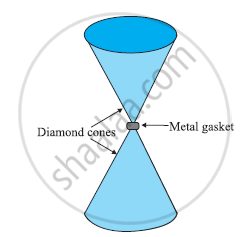Q 18 | Page 245

A rod of length 1.05 m having negligible mass is supported at its ends by two wires of steel (wire A) and aluminium (wire B) of equal lengths as shown in Figure. The cross-sectional areas of wires A and B are 1.0 mm2 and 2.0 mm2, respectively. At what point along the rod should a mass be suspended in order to produce (a) equal stresses and (b) equal strains in both steel and aluminium wires.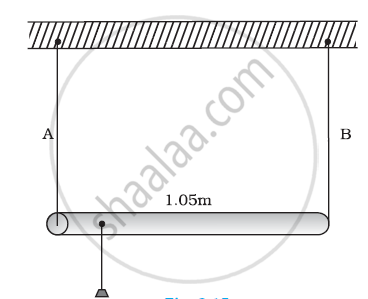Q 19 | Page 245

A mild steel wire of length 1.0 m and cross-sectional area 0.50 × 10–2 cmis stretched, well within its elastic limit, horizontally between two pillars. A mass of 100 g is suspended from the mid-point of the wire. Calculate the depression at the midpoint.

Q 20 | Page 245

Two strips of metal are riveted together at their ends by four rivets, each of diameter 6.0 mm. What is the maximum tension that can be exerted by the riveted strip if the shearing stress on the rivet is not to exceed 6.9 × 107 Pa? Assume that each rivet is to carry one-quarter of the load.

Q 21 | Page 245

The Marina trench is located in the Pacific Ocean, and at one place it is nearly eleven km beneath the surface of the water. The water pressure at the bottom of the trench is about 1.1 × 108 Pa. A steel ball of initial volume 0.32 m3 is dropped into the ocean and falls to the bottom of the trench. What is the change in the volume of the ball when it reaches to the bottom?

## Chapter 9: Mechanical Properties of Solids## NCERT solutions for Class 11 Physics Textbook chapter 9 - Mechanical Properties of Solids

NCERT solutions for Class 11 Physics Textbook chapter 9 (Mechanical Properties of Solids) include all questions with solution and detail explanation. This will clear students doubts about any question and improve application skills while preparing for board exams. The detailed, step-by-step solutions will help you understand the concepts better and clear your confusions, if any. Shaalaa.com has the CBSE Class 11 Physics Textbook solutions in a manner that help students grasp basic concepts better and faster.

Further, we at Shaalaa.com provide such solutions so that students can prepare for written exams. NCERT textbook solutions can be a core help for self-study and acts as a perfect self-help guidance for students.

Concepts covered in Class 11 Physics Textbook chapter 9 Mechanical Properties of Solids are Stress-strain Curve, Stress and Strain, Hooke’s Law, Young’s Modulus, Determination of Young’s Modulus of the Material of a Wire, Shear Modulus, Bulk Modulus, Applications of Elastic Behaviour of Materials, Elastic Energy, Elastic Behaviour of Solid.

Using NCERT Class 11 solutions Mechanical Properties of Solids exercise by students are an easy way to prepare for the exams, as they involve solutions arranged chapter-wise also page wise. The questions involved in NCERT Solutions are important questions that can be asked in the final exam. Maximum students of CBSE Class 11 prefer NCERT Textbook Solutions to score more in exam.

Get the free view of chapter 9 Mechanical Properties of Solids Class 11 extra questions for Class 11 Physics Textbook and can use Shaalaa.com to keep it handy for your exam preparation

Advertisement Remove all ads
Share
Notifications

View all notifications

Forgot password?